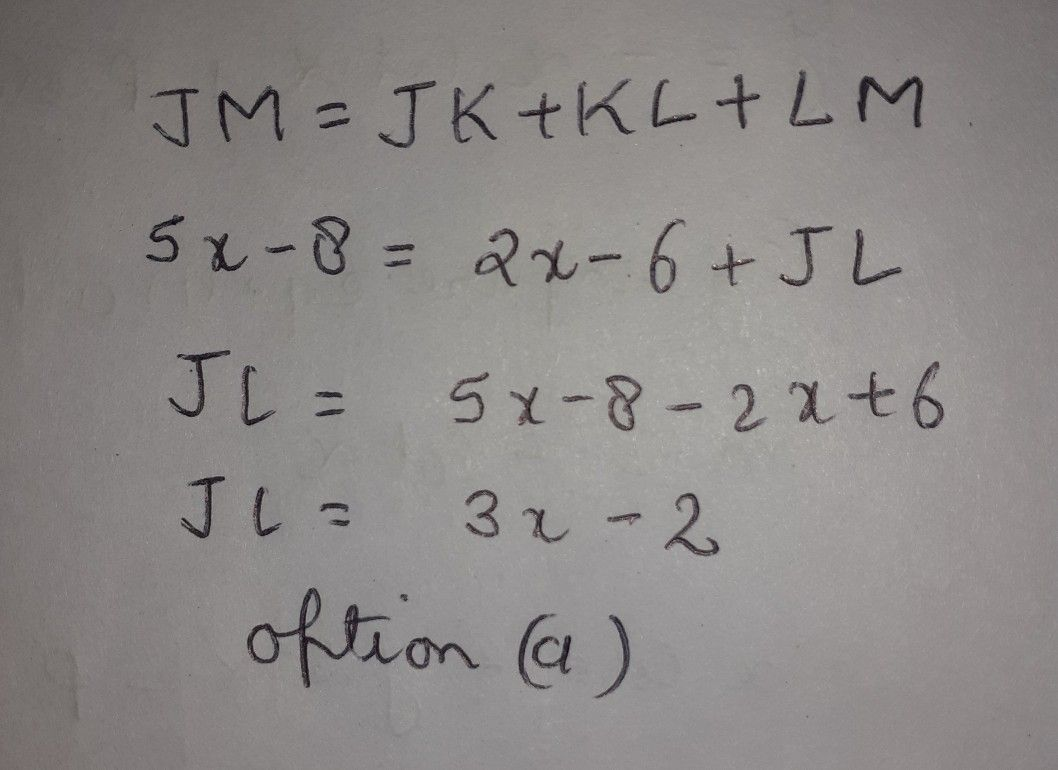Symbol
Problem$y$ $9$ $3$ $11\right)M=5x-8$ and $\left(M=2x-6$ which expression represents JL? $k$ $L$ $A$ $○$ $3x-2$ $O$ $3x-14$ $O$ $7x-2$ $0$ $7x-14$
10th-13th grade
Geometry
SolutionQanda teacher - AnandGuruare you satisfied with my answer
please evaluate my answer and give me gift coins
also follow me for more answers